# “Deep Residual Learning for Image Recognition” Summarized

Categories:

Updated:

https://arxiv.org/abs/1512.03385 (2015-12-10)

## 1. Deep Networks

Deep networks naturally integrate low/mid/high-level features and classifiers in an end-to-end multi-layer fashion, and the “levels” of features can be enriched by the number of stacked layers.

But as networks get deeper, vanishing/exploding gradients problems appear. They hamper convergence. To solve these problems, normalized initialization and intermediate normalization layers were introduced.

Nevertheless, adding more layers to a stuiably deep models leads to higher ‘training error’ This problem is called ‘degradation’. Note that ‘training error’ degrades so it is not caused by overfitting.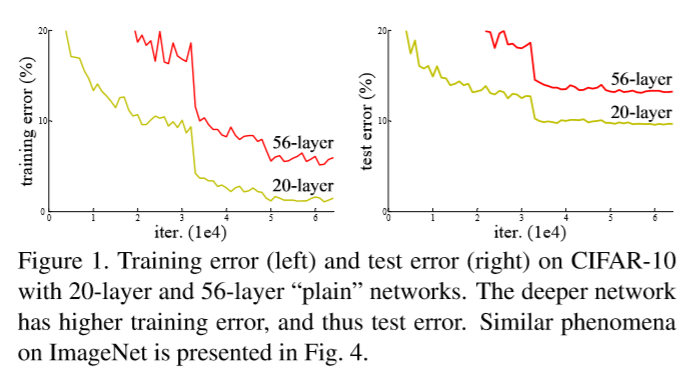## 2. Residual Learning

The authors let the layers fit a residual mapping $F(x) := H(x)-x$, where the original mapping is $H(x)$. So the original mapping is recast into $F(x)+x$.Those connections which skip one or more layers is called ‘shortcut connections’ or ‘skip connections’. Shortcut connections simply perform identity mapping and their outputs are added to the outputs of the block(stacked layers). Identity shrotcut connections add neither extra parameter nor computational complexity.

The degradation problem suggests that the solvers might have difficulties in approximating identity mappings by multiple nonlinear layers. However with the residual learning reformulation, if identity mappings are optimal, the solvers may simply drive the weights of the multiple nonlinear layers toward zero to approach identity mappings.

## 3. Dimension Match

The dimensions of $x$ and $F$ must be equal. If this is not the case(e.g. when changing the input/output channels), we have two ways.

First, we can perform a linear projection $W_s$ by the shortcut connections. This is done by 1x1 convolution. $y = F(x, {W_i}) + W_sx$

Second, the shrotcut can still perform identity mapping with extra zero padding for increasing dimensions.

## 4. Network Architectures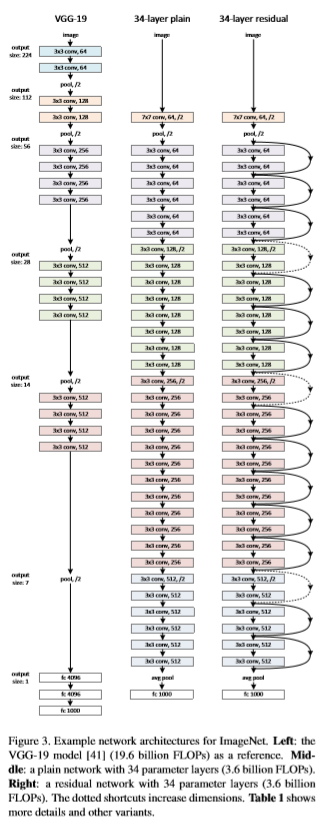### 1. Plain Network

Plain baselines are mainly inspered by VGG nets and have two simple design rules.

1. for the same output feature map size, the layers have the same number of filters.
2. if the feature map size is halved, the number of filters is doubled so as to preserve the time complexity per layers.

### 2. Residual Network

For every two or three layers, shortcut connections are added. If the dimensions match, identity shortcuts are directly used. If dimensions don’t match, the authors inserted zero entries (case A) or 1x1 convolutions (case B).

### 3. Bottleneck for Deeper Nets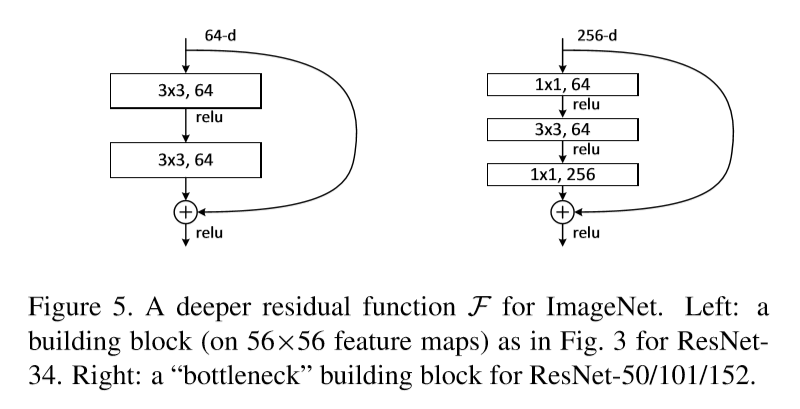For deeper nets, long training time is concerned. To save time, ‘bottleneck’ layer is introduced.

For each residual function F, stack of 3 layers instead of 2 is used. The three layers are 1x1, 3x3, and 1x1 convolutions, where the 1x1 layers are responsible for reducing and then increasing(restoring) dimensions, leaving the 3x3 layera bottlenceck with smaller input/output dimensions.

If projection shortcut is used instead of identity shortcut, the time complexity and model size are doubled, as the shortcut is connected to the two high-dimensional ends. So identity shortcuts lead to more efficient models for the bottleneck designs.

50-layer ResNet is constructed by replacing 2-layer block with 3-layer bottleneck block, and 101-layer / 152-layer ResNets are constructed by using more 3-layer blocks.

## 5. Implementation

• The image is resized with its shorter side randomly sampled in [256, 480] for scale augmentation.
• A 224x224 crop is randomly sampled from an image or its horizontal flip
• per-pixel mean subtracted
• standard color augmentation
• batch normalization right after each convolution and before activation
• SGD with 0.0001 weight decay and 0.9 momentum (0.01 lr to warmup the training until the training error is below 80% then go back to 0.1 lr in case of 110-layer ResNet with Cifar10)
• mini-batch size of 256
• learning rate starts from 0.1 and is divided by 10 when the error plateaus
• trained for up to $60\times 10^4$ iterations

## 6. Imagenet Classification Experiments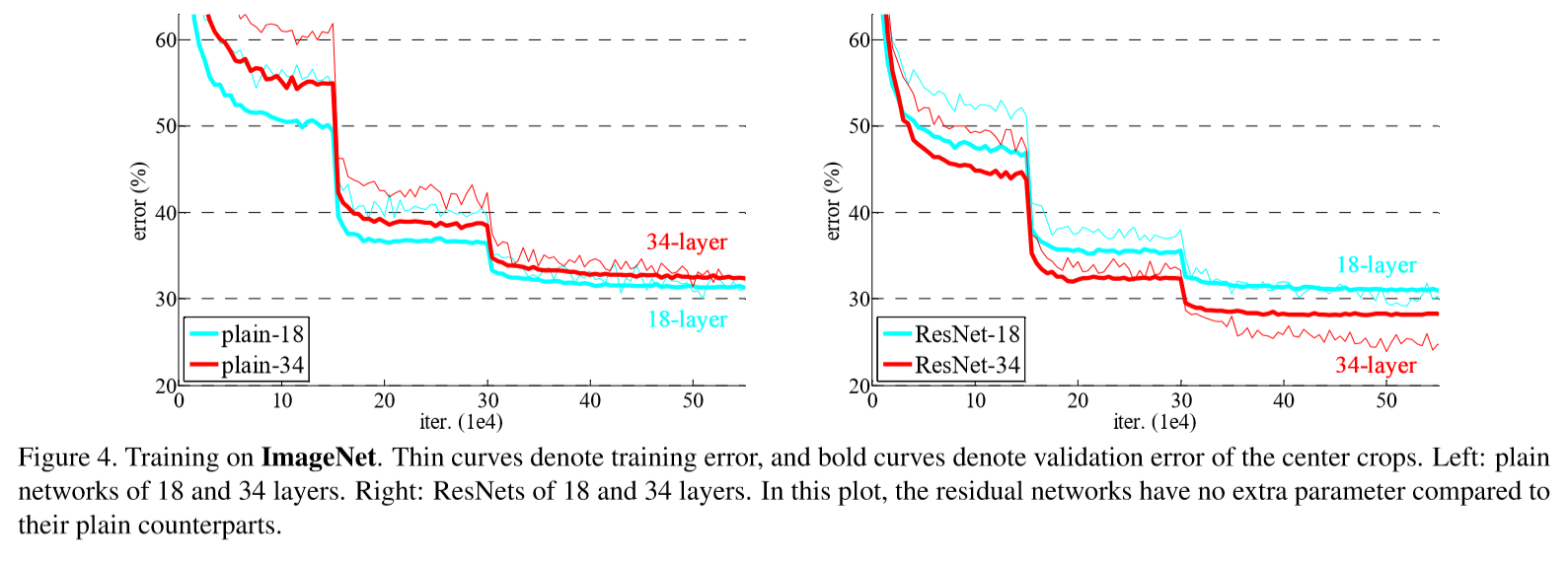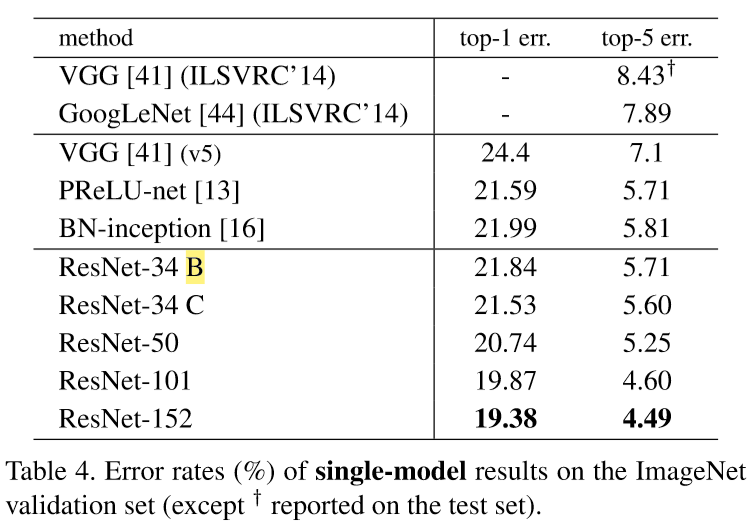### 1. Plain Networks

Deeper 35-layer plain net has higher validation error than the shallower 18-layer plain net. This is likely casued by degradation.

The authors say that this optimization difficulty is ‘unlikely’ to be caused by vanishing gradients, because they used BN(Batch Normalization) and also verified that the backward propagated gradients exhibit healthy norms so that neither forward nor backward signals vanish.

They conjecture that the deep plain nets may have exponentially low convergence rates, which impact the reducing of the training error.

### 2. Residual Networks

34-layer ResNet exhibits considerably lower training error and is generalizble to the validation data. This indicates that the degradation problem is well addressed in this setting and it managed to obtain accuracy gains from increased depth.

Also, both 18-layer plain/residual nets are comparably accurate, but the 18-layer ResNet converges faster.

For identity vs projection shortcuts, they’ve experimented three cases. (A) zero-padding for increasing dimensions(all shortcuts are parameter-free) (B) projection shortcuts for increasing dimensions (C) all shortcuts are projections. B is slightly better than A because zero-padding dimensions in A couldn’t merit from residual learning. C is marginally better than B, but the authors attributed this to the extra parameters introduced, and not to the residual learning. So the authors continued with options A or B.

By the way, testing result of 1202-layer network is worse than 110-layer network because of overfitting.

### Cifar-10 Experiment Result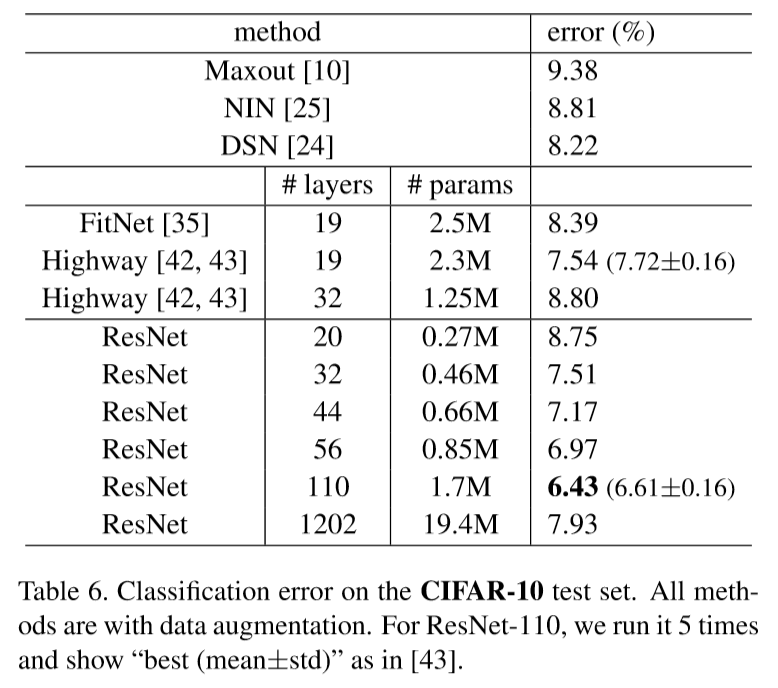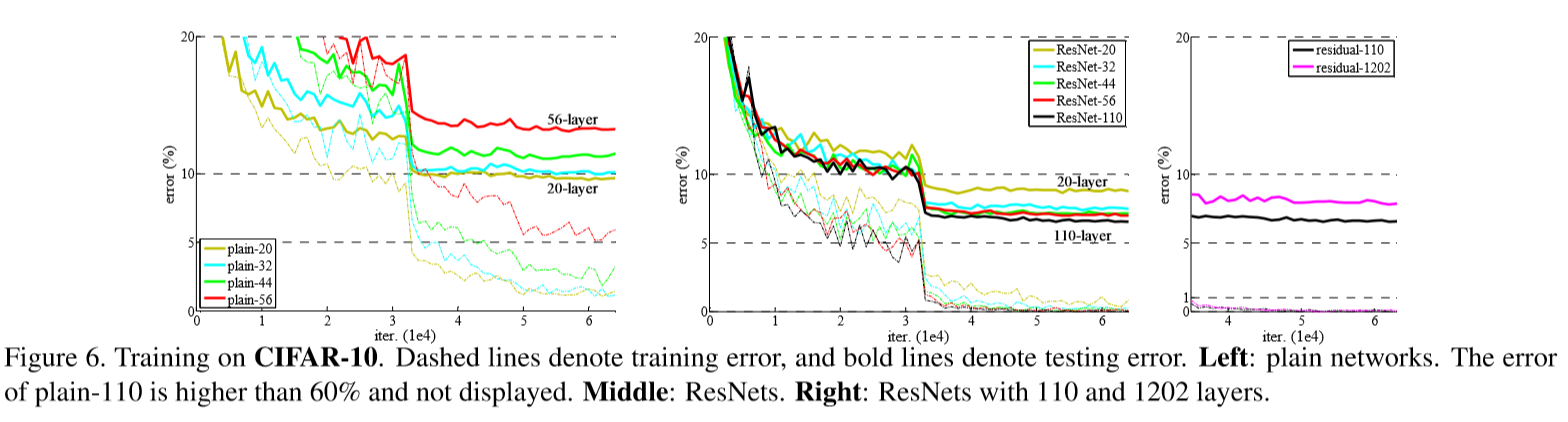### PASCAL VOC, COCO Result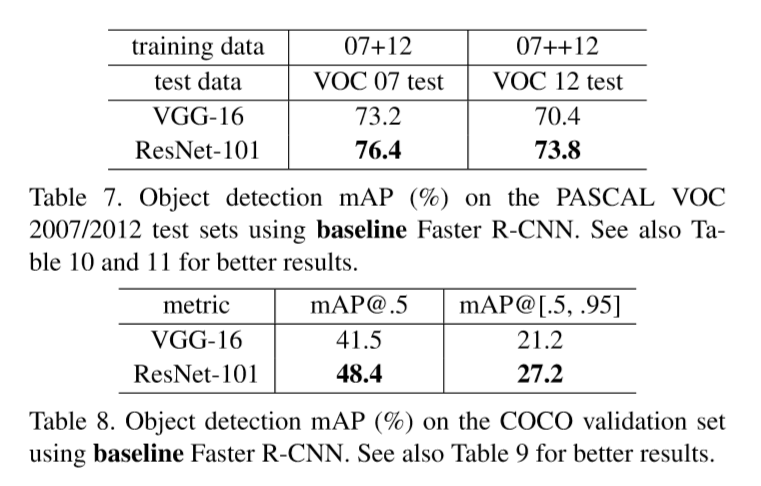## 7. Analysis of Layer Responses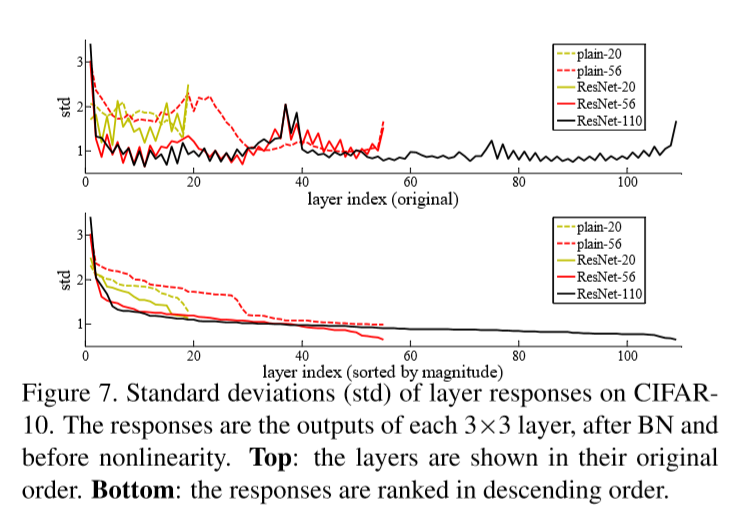ResNets have generally smaller responses than their plain counterparts. These results support the idea that the residual functions might be generally closer to zero than the non-residual functions.

Also, deeper ResNet has smaller magnitudes of responses, because when there are more layers, an individual layer of ResNets tends to modify the signal less.

Categories: